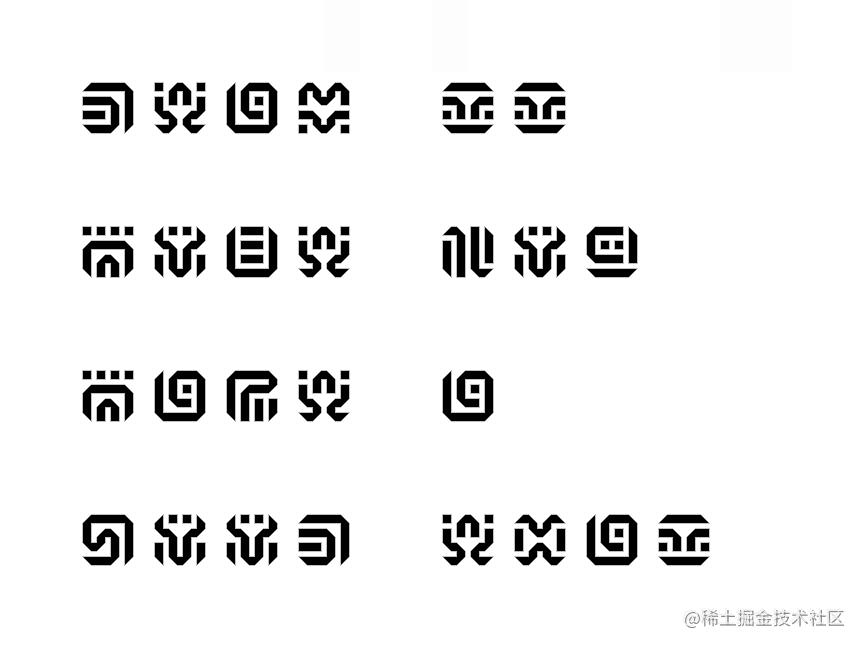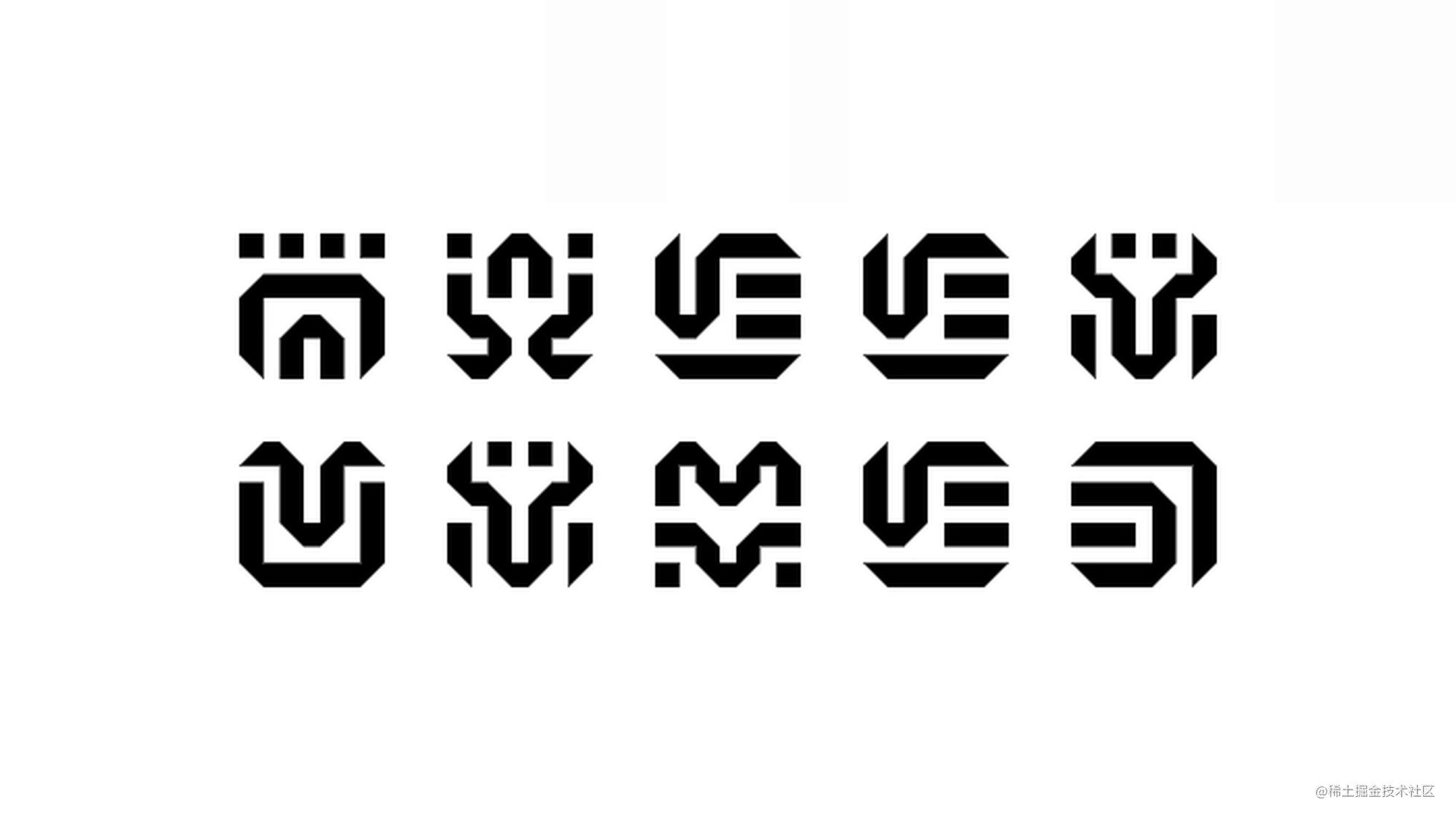# 摸一个塞尔达希卡文字转换器

## 希卡族文字转换器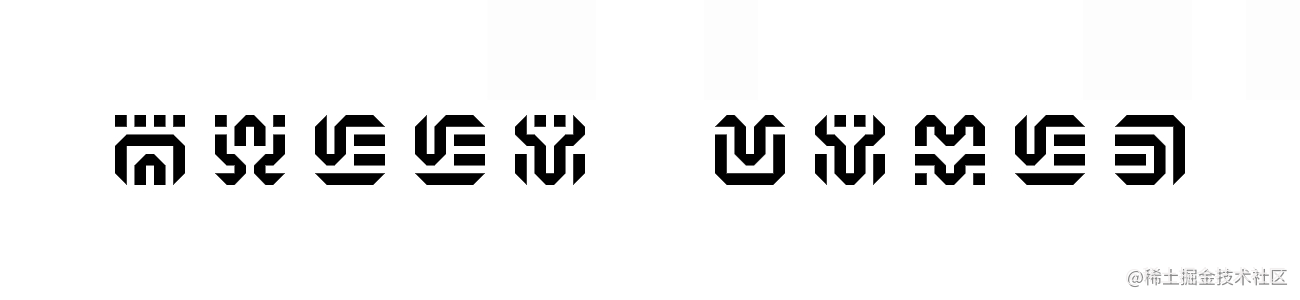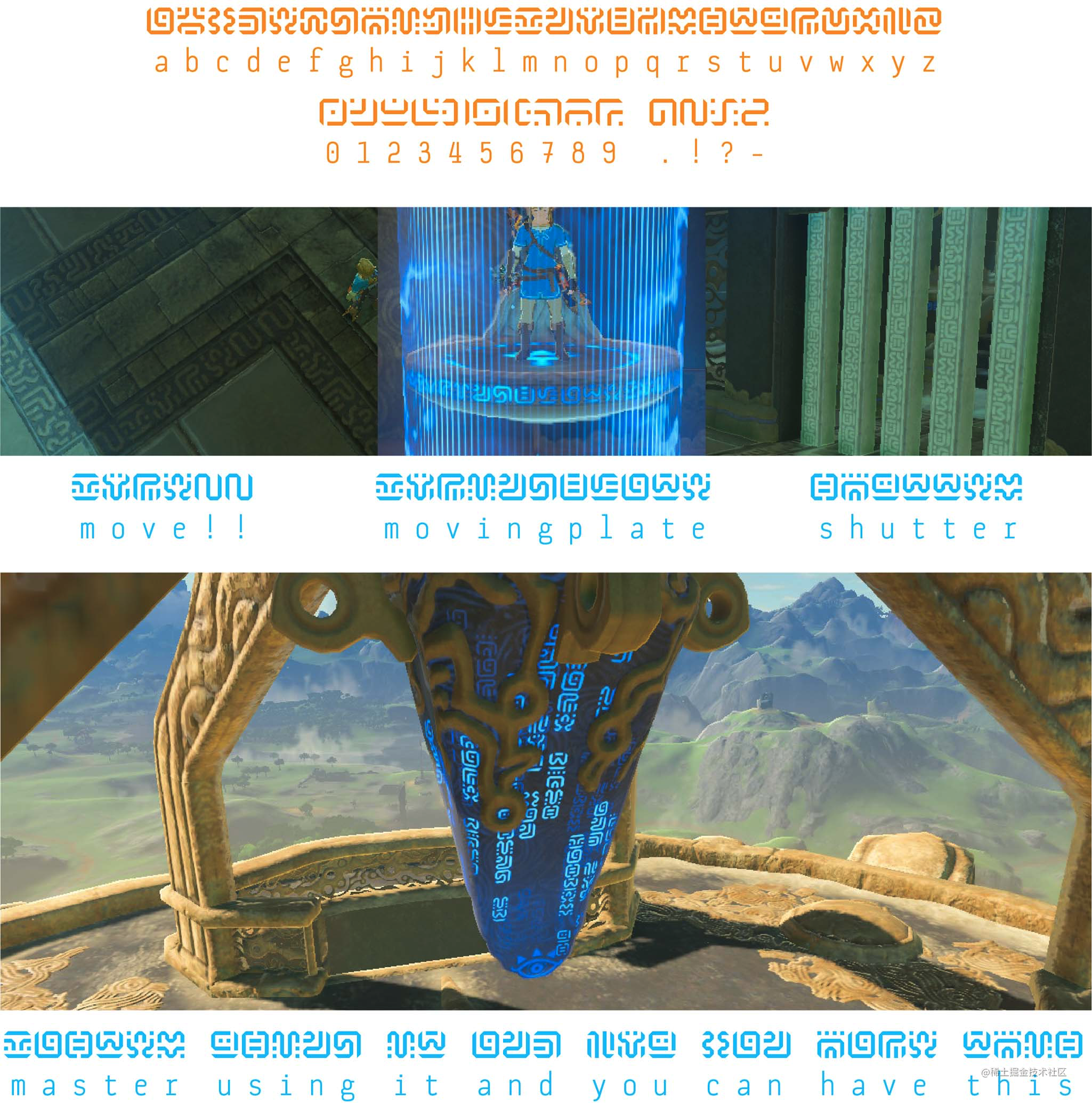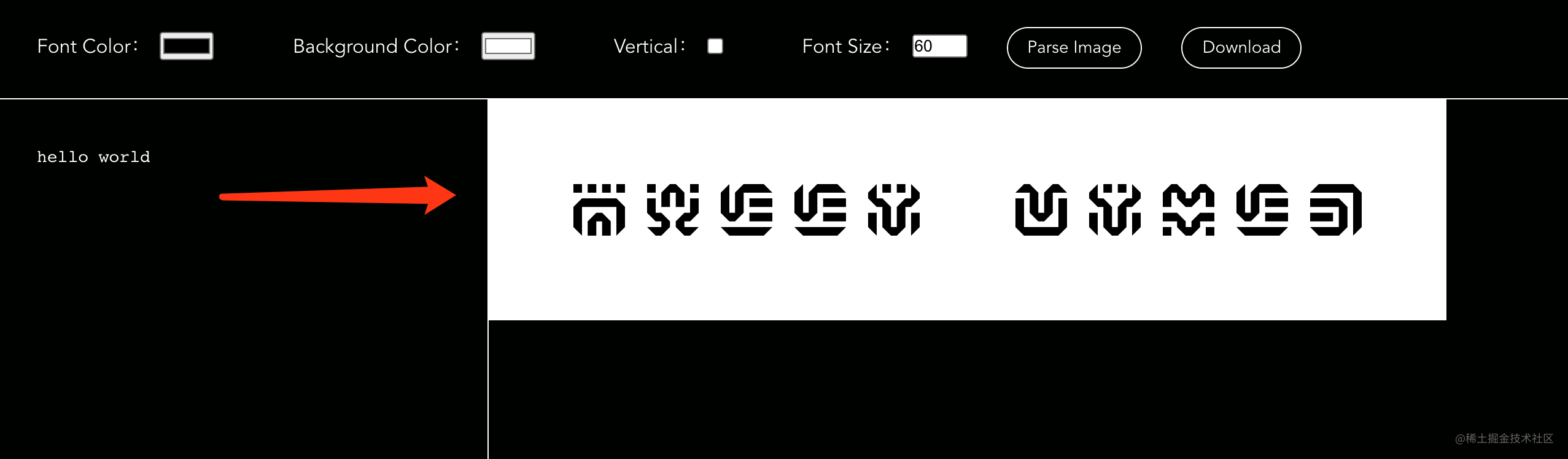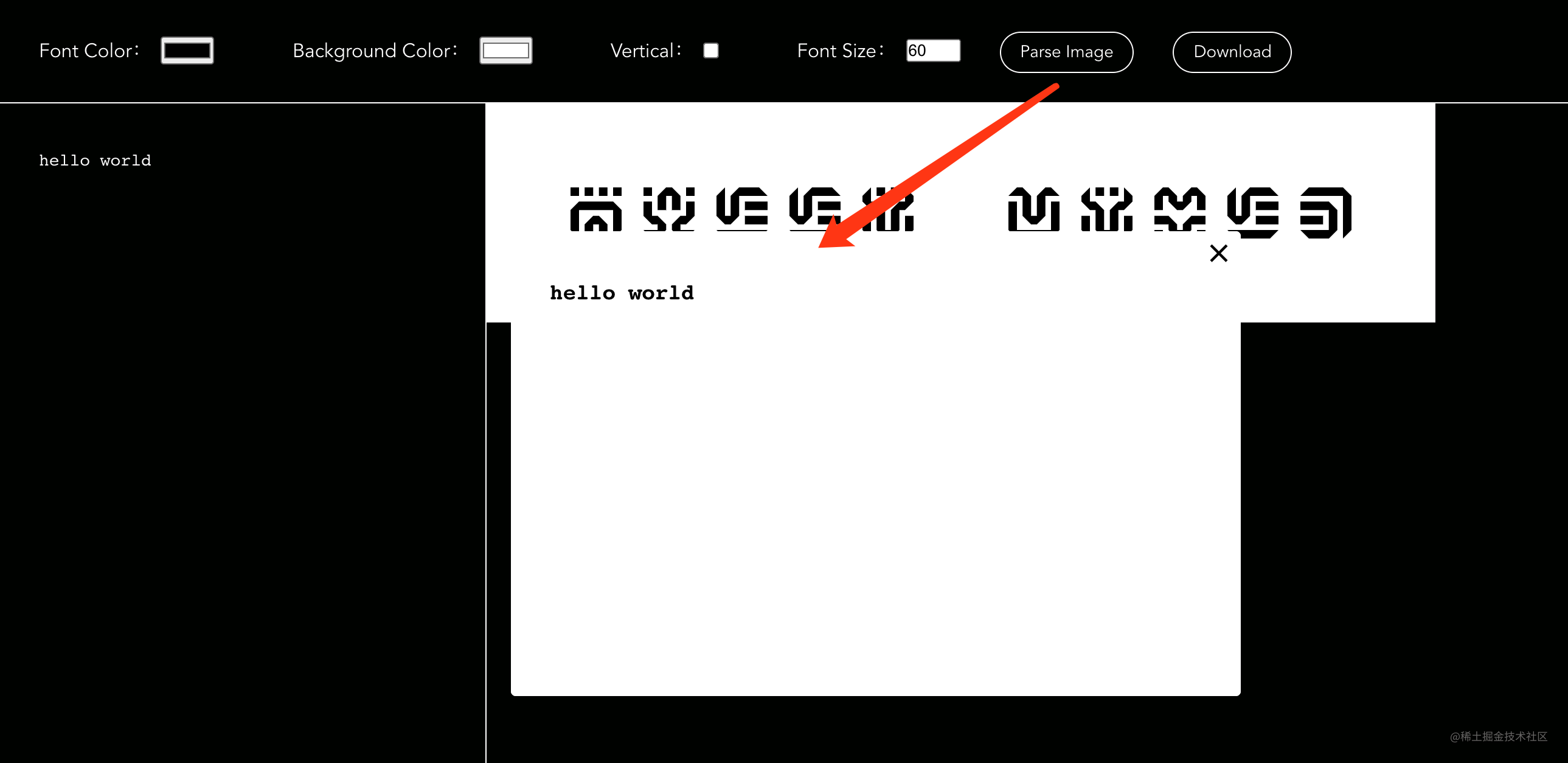## 英文 -> 希卡文转化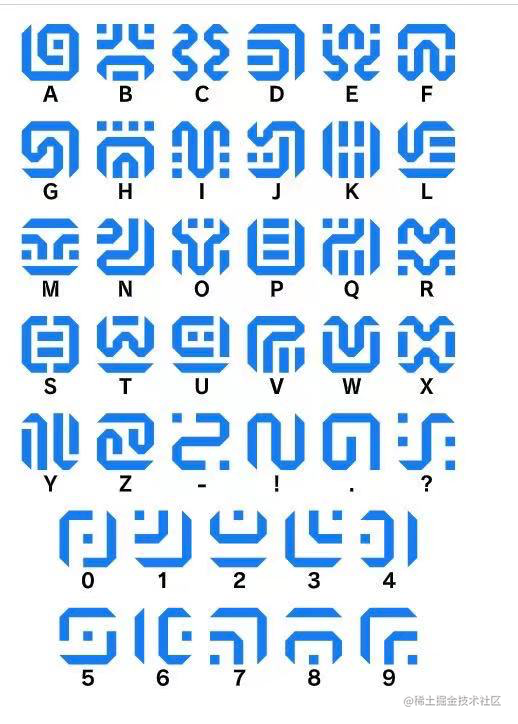3type.cn/css/fonts/s…

``````@font-face {
font-family: "SheikahGlyphs";
src: url("https://3type.cn/css/fonts/sheikahglyphs-regular-webfont.woff2") format("woff2");
}

.sheikah-word {
font-family: SheikahGlyphs;
}

``````<span class="sheikah-word">abc</span>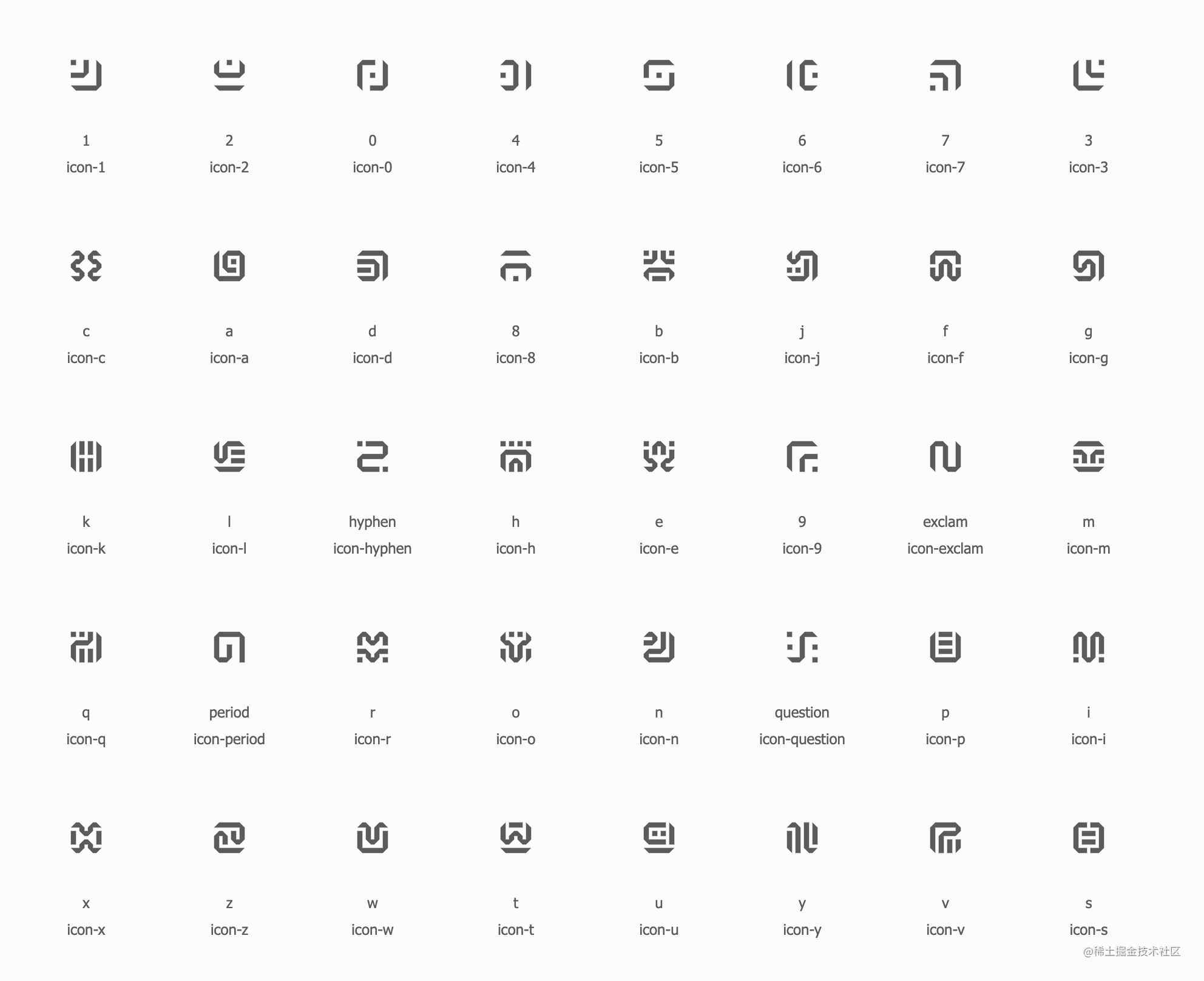``````<script src="//at.alicdn.com/t/font_2375469_s4wmtifuqro.js"></script>

``````<template>
<svg
class="word-icon"
aria-hidden="true"
:style="iconStyle"
>
</svg>
</template>

<script>
import { computed } from 'vue';

export default {
name: 'WordIcon',

props: {
// 图标名称
name: {
type: String,
required: true,
},

width: {
type: Number,
default: '',
},

height: {
type: Number,
default: '',
},

color: {
type: String,
default: '',
},

opacity: {
type: String,
default: '',
},
},

setup: (props) => {
const iconName = computed(() => props.name ? `#icon-\${props.name}` : '');
const iconStyle = computed(() => ({
color: props.color,
opacity: props.opacity,
width: `\${props.width}px`,
height: `\${props.height}px`,
}));
return {
iconName,
iconStyle,
};
},
};
</script>

<style>
.word-icon {
overflow: hidden;
width: 1em;
height: 1em;
margin: 0;
fill: currentColor;
}
</style>

``````<template>
<section
class="words-panel"
ref="container"
>
<div
class="words-panel__groups"
v-for="(words, index) in wordGroups"
:key="index"
>
<WordIcon
class="words-panel__icon"
v-for="(word, idx) in words"
:key="idx"
:name="word"
:width="size"
:height="size"
>
{{ word }}
</WordIcon>
</div>
</section>
</template>

<script>
import { computed, ref } from 'vue';
import WordIcon from './icon.vue';

const WORDS = [
'0', '1', '2', '3', '4', '5', '6', '7', '8', '9',
'a', 'b', 'c', 'd', 'e', 'f', 'g', 'h', 'i', 'j',
'k', 'l', 'm', 'n', 'o', 'p', 'q', 'r', 's', 't',
'u', 'v', 'w', 'x', 'y', 'z', '.', '!', '?', '-',
];

export default {
name: 'WordsPanel',

components: {
WordIcon,
},

data() {
return {
words: 'hello world',
size: 60,
};
},

setup: (props) => {
const container = ref(null);
const wordGroups = computed(() => {
return props.words
.toLowerCase()
.split('\n')
.map(words => words.split('').map(v => WORDS.includes(v) ? v : ''));
});

return {
container,
wordGroups,
};
},
};
</script>

``````(async function () {
const svg =
`<svg viewBox="0 0 200 200" xmlns="http://www.w3.org/2000/svg">
<foreignObject x="0" y="0" width="200" height="200">
<div xmlns="http://www.w3.org/1999/xhtml">OUTPUT</div>
</foreignObject>
</svg>`;

const dataUrl = 'data:image/svg+xml;charset=utf-8,' + svg;

const loadImage = (url) => {
return new Promise((resolve, reject) => {
const img = new Image();
img.onerror = (e) => reject(e);
img.src = url;
});
};

const canvas = document.createElement('canvas');
canvas.width = 120;
canvas.height = 60;
const ctx = canvas.getContext('2d');
ctx.drawImage(image, 0, 0);
console.log(canvas.toDataURL());
})();

dom-to-image 内部处理与上面的流程类似，处理我们的字体图标时会生成类似于如下的 SVG 结构：

``````<svg xmlns="http://www.w3.org/2000/svg">
<foreignObject width="120" height="60">
<svg>
</svg>
</foreignObject>
</svg>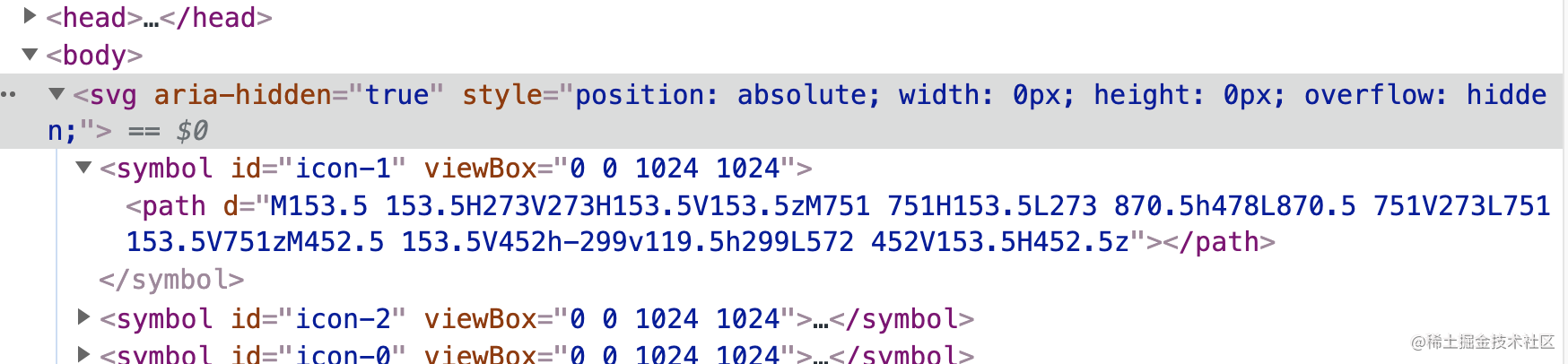``````<svg xmlns="http://www.w3.org/2000/svg">
<foreignObject width="120" height="60">
<svg>
<symbol id="icon-a" viewBox="0 0 1024 1024">
<path d="xxx"></path>
</symbol>
</svg>
</foreignObject>
</svg>

``````import domtoimage from 'dom-to-image';

// fix 节点中 svg 图标依赖
function fixSvgIconNode(node) {
if (node instanceof SVGElement) {
const useNodes = Array.from(node.querySelectorAll('use') || []);
useNodes.forEach((use) => {
// 将 svg 图片中依赖的 <symbol> 节点塞到当前 svg 节点下
if (id && !node.querySelector(id)) {
const symbolNode = document.querySelector(id);
if (symbolNode) {
node.insertBefore(
symbolNode.cloneNode(true),
node.children
);
}
}
});
}
return true;
}

export default function exportImage (node) {
return domtoimage.toPng(node, { filter: fixSvgIconNode })
.then(dataUrl => {
console.log(dataUrl);
});
}

## 希卡文 -> 英文转化

### 图片盲水印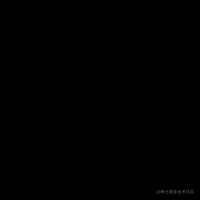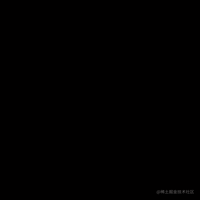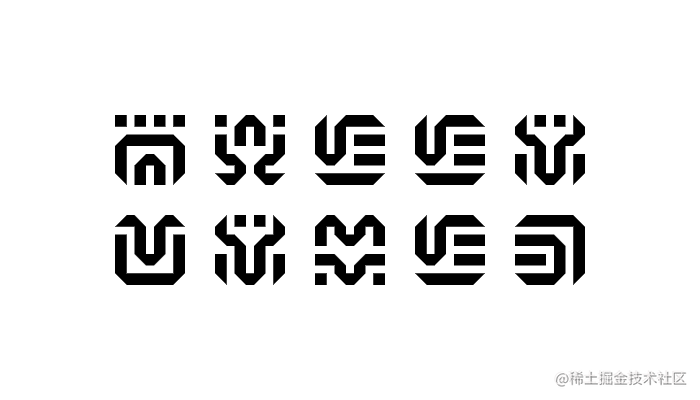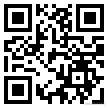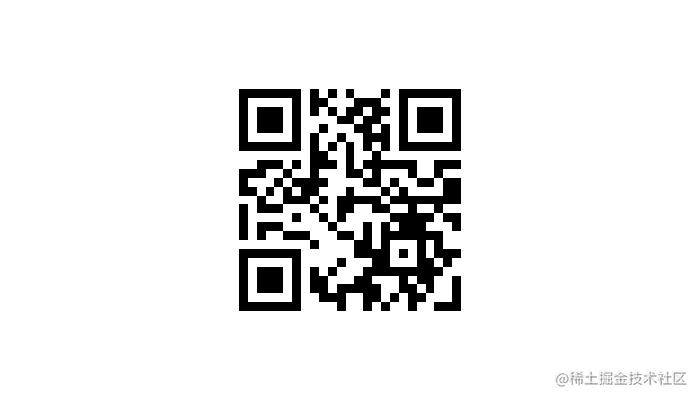``````// 写入二维码水印
function writeMetaInfo(baseImageData, qrcodeImageData) {
const { width, height, data } = qrcodeImageData;
for (let x = 0; x < width; x++) {
for (let y = 0; y < height; y++) {
// 选用 r 通道来隐藏信息
const r = (x + y * width) * 4;
const v = data[r];
// 二维码白色部分（背景）标识为 1，黑色部分（内容）标识为 0
const bit = v === 255 ? 1 : 0;
// 如果当前 R 通道色值奇偶性和二维码对应像素不一致则进行加减一使其奇偶性一致
if (baseImageData.data[r] % 2 !== bit) {
baseImageData.data[r] += bit ? 1 : -1;
}
}
}
return baseImageData;
}

// 读取二维码水印
const { width, height, data } = imageData;
const qrcodeImageData = new ImageData(width, height);
for (let x = 0; x < width; x++) {
for (let y = 0; y < height; y++) {
// 读取 r 通道息
const r = (x + y * width) * 4;
// 奇数颜色为白色 255，偶数颜色为黑色 0
const v = data[r] % 2 === 0 ? 0 : 255;
qrcodeImageData.data[r] = v;
qrcodeImageData.data[r + 1] = v;
qrcodeImageData.data[r + 2] = v;
qrcodeImageData.data[r + 3] = 255;
}
}
return qrcodeImageData;
}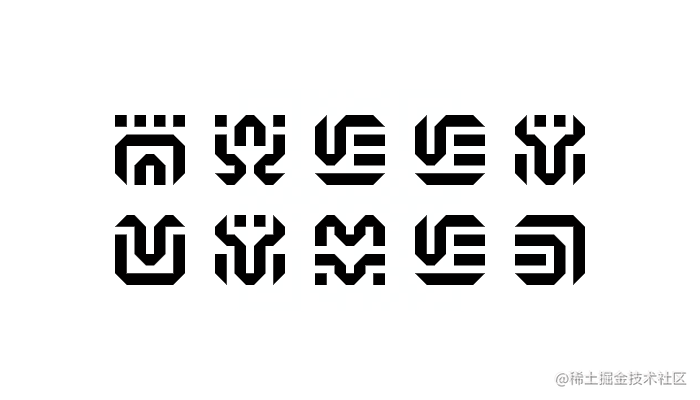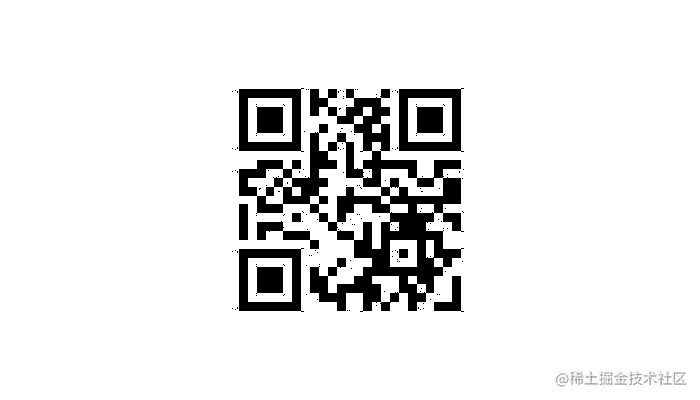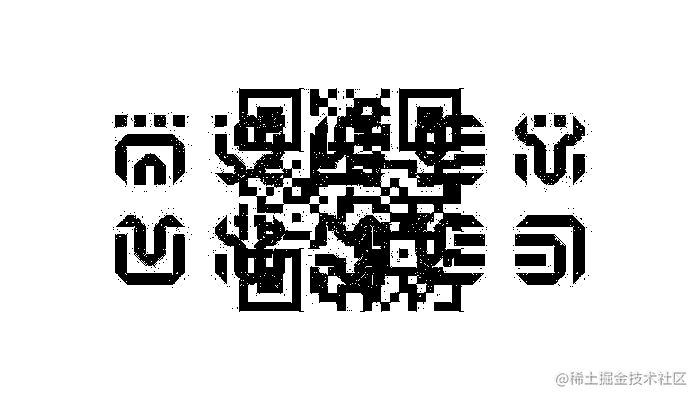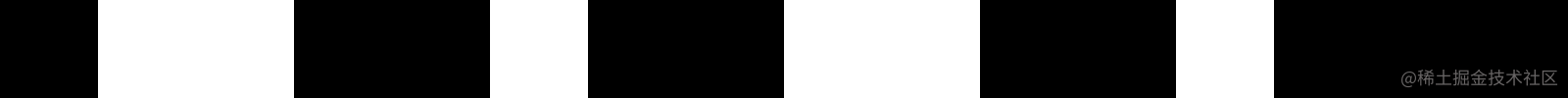``````[100, 200]
[01100100, 11001000]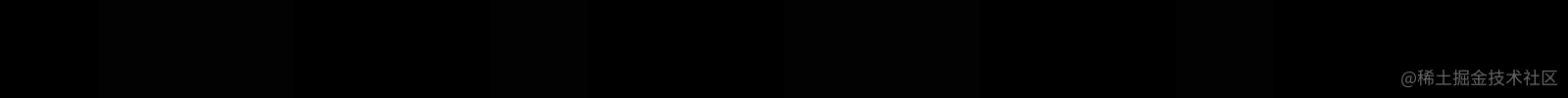``````// 统一成 8 位
return ('00000000' + bits).slice(-8);
}

function write(data) {
const bits = data.reduce((s, it) => s + paddingLfet(it.toString(2)), '');
const size = 100;
const width = size * bits.length;
const canvas = document.createElement('canvas');
canvas.width = width;
canvas.height = size;
const ctx = canvas.getContext('2d');
ctx.fillStyle = '#0000000';
ctx.fillRect(0, 0, width, size);
for (let i = 0; i < bits.length; i++) {
if (Number(bits[i])) {
ctx.fillStyle = '#020202';
ctx.fillRect(i * size, 0, size, size);
}
}
return canvas.toDataURL();
}

const canvas = document.createElement('canvas');
canvas.width = image.naturalWidth;
canvas.height = image.naturalHeight;
const ctx = canvas.getContext('2d');
ctx.drawImage(image, 0, 0);
const size = 100;
const bits = [];
for (let i = 0; i < 16; i++) {
const imageData = ctx.getImageData(i * size, 0, size, size);
const r = imageData.data;
const g = imageData.data;
const b = imageData.data;
bits.push(r + g + b === 0 ? 0 : 1);
}
return bits;
}

### 相似图片识别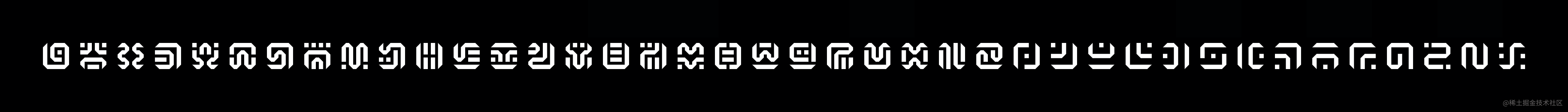• 格子的大小
• 标准的希卡符号字典图片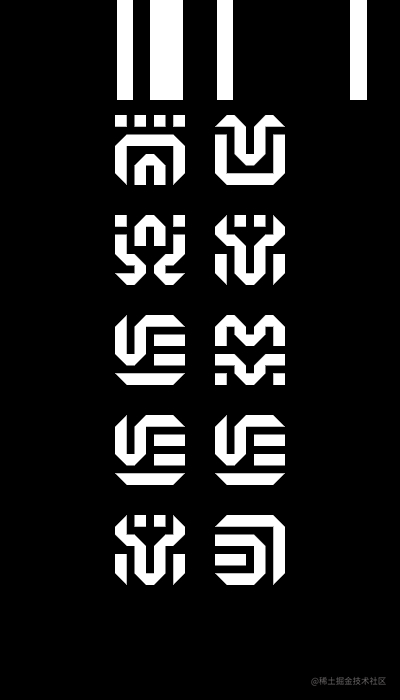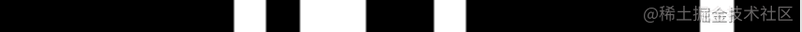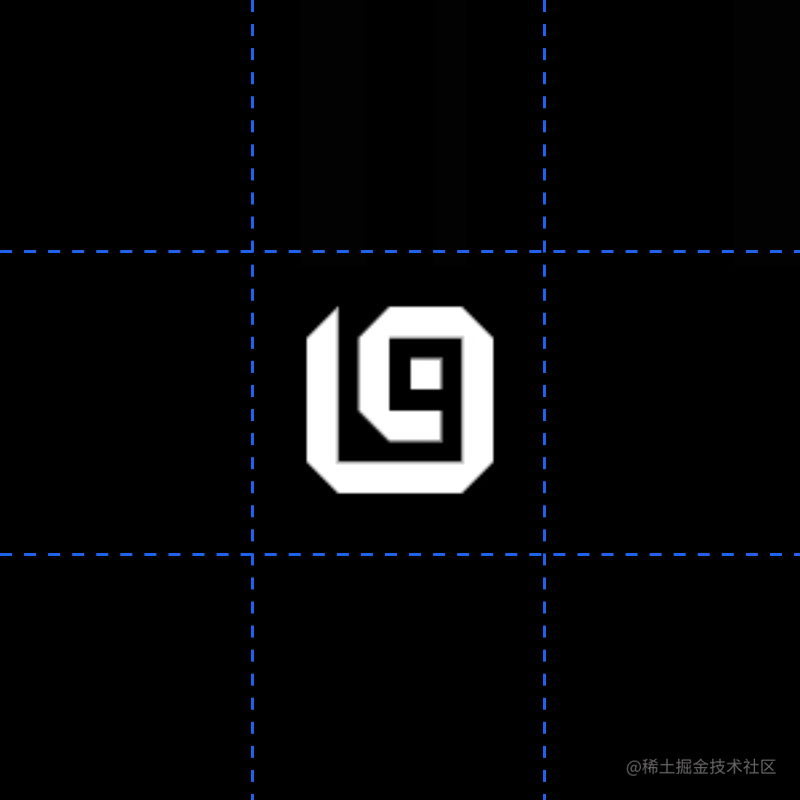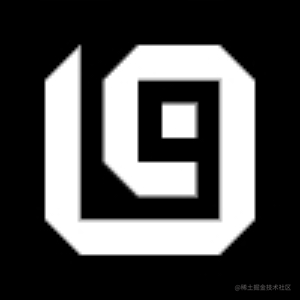``````00101101
00111001

• 将图片缩成 8 x 8 的大小
• 对图片进行灰度处理
• 对图片进行二值化处理（统一成黑白可以映射成 01）
• 输出图片指纹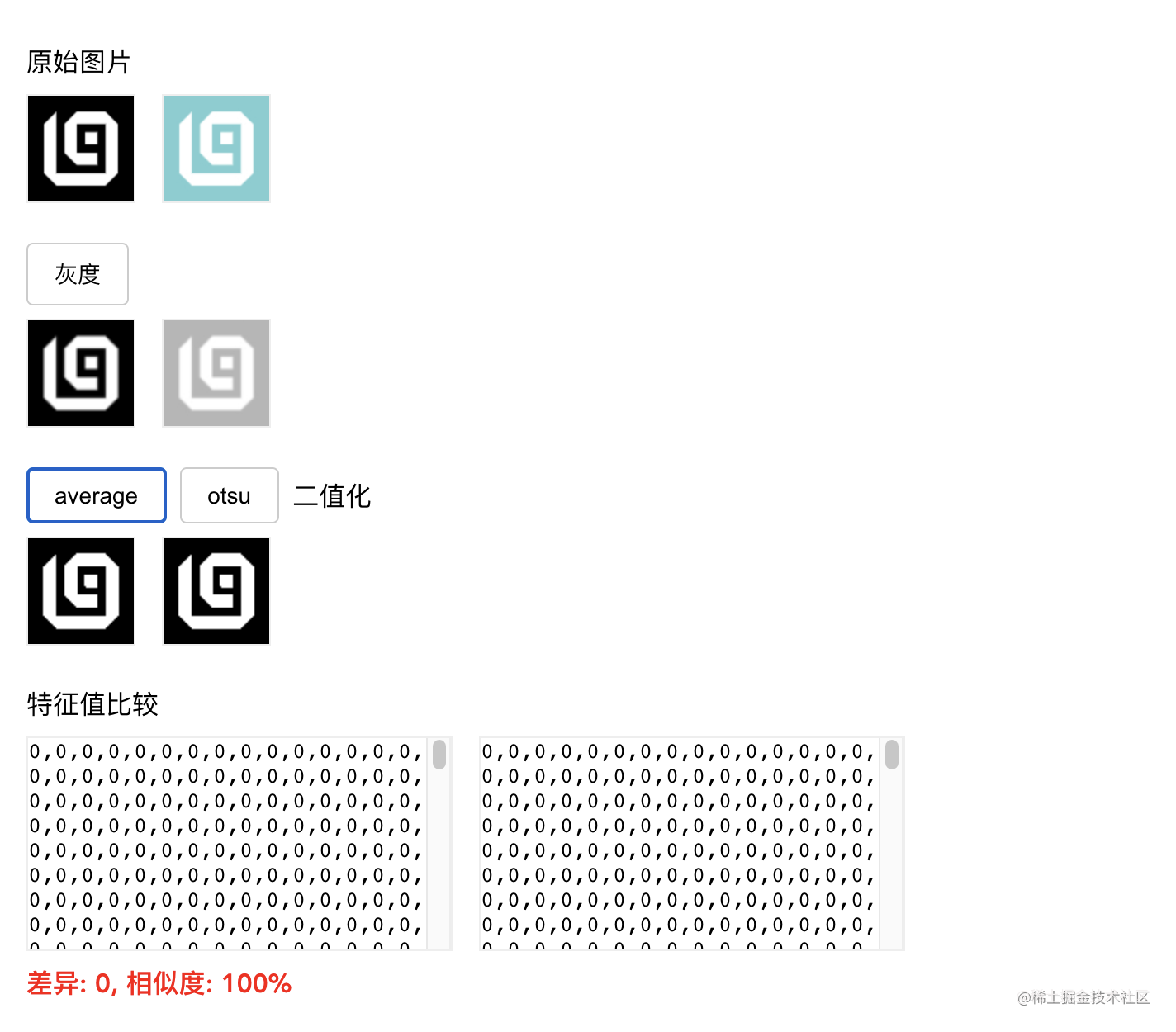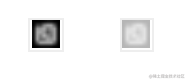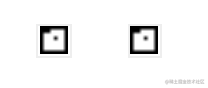## 其他